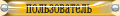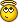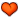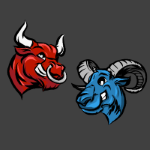[ Новые сообщения · Игроделы · Правила · Поиск ]
 Форум игроделов » Конструкторы игр и лёгкие в освоении системы разработки игр » Game Maker » Враги не наносят урон при ударе (gamemaker studio 2, rpg, вид сверху)
Враги не наносят урон при ударе
 pathra Дата: Среда, 06 Февраля 2019, 18:04 | Сообщение # 1 уже былСейчас нет на сайте враги при столкновении с игроком создают объект obj_enemy_damage, и он создается, но урон не наноситсяcreate:Кодspeed_enemy = 0.8;phy_fixed_rotation = true;hp = 3;R = 150;moveToPl = 0;maxatksp = 30;atksp = 0;dmg = 1;step:Кодif moveToPl!=1 and obj_player.y<(self.y + R) and obj_player.y>(self.y - R) and obj_player.x<(self.x + R) and obj_player.x>(self.x - R){    moveToPl = 1;}if moveToPl == 1{    phy_position_x += sign(obj_player.x-x) * speed_enemy;    phy_position_y += sign(obj_player.y-y) * speed_enemy;}depth = -y;if atksp!=maxatksp{    atksp+=1;}if hp<=0{    instance_create_depth(x,y,0,obj_enemy_death);    instance_destroy();}collision с obj_player:Кодif atksp == maxatksp{    var damage_1 = instance_create_depth(x,y,0,obj_enemy_damage);    damage_1.creator = id;    atksp = 0;}У объекта obj_enemy_damagecreate:Кодknockb = 10;creator = noone;alarm = 1;collision с obj_player:Кодif (other.id != creator){    other.hp -= creator.dmg;        var dir = point_direction(creator.x,creator.y, other.x, other.y);    var x_force = lengthdir_x(knockb, dir);    var y_force = lengthdir_y(knockb, dir);        with(other){  physics_apply_impulse(x,y,x_force,y_force);    }}alarm:Кодinstance_destroy();и наконец у самого obj_playercreate:Кодphy_fixed_rotation = true;speed_player = 3;image_speed = 0;hp = 7;maxatksp = 30;atksp = 0;dmg = 1;step:Кодvar right_move = (keyboard_check(vk_right) or keyboard_check(ord("D")))var left_move = (keyboard_check(vk_left) or keyboard_check(ord("A")))var up_move = (keyboard_check(vk_up) or keyboard_check(ord("W")))var down_move = (keyboard_check(vk_down) or keyboard_check(ord("S")))if (up_move) {    phy_position_y -=speed_player;    sprite_index = player_sprites_up;    image_speed = 1;}if (down_move) {    phy_position_y +=speed_player;    sprite_index = player_sprites_down;    image_speed = 1;}if (right_move) {    phy_position_x +=speed_player;    sprite_index = player_sprites_right;    image_speed = 1;}if (left_move) {    phy_position_x -=speed_player;    sprite_index = player_sprites_left;    image_speed = 1;}if (!right_move and !left_move and !up_move and !down_move){    image_speed = 0;    image_index = 0;}depth = -y;if hp<=0    instance_destroy();    if atksp!=maxatksp{    atksp+=1;}    if keyboard_check_pressed(vk_space) and atksp==maxatksp{    if self.sprite_index == player_sprites_right{   var damage_1 = instance_create_depth(x+20,y, 0, obj_player_damage);   damage_1.creator = id;    }  if self.sprite_index == player_sprites_left{   var damage_1 = instance_create_depth(x-20,y, 0, obj_player_damage);   damage_1.creator = id;    }  if self.sprite_index == player_sprites_up{   var damage_1 = instance_create_depth(x,y-20, 0, obj_player_damage);   damage_1.creator = id;    }  if self.sprite_index == player_sprites_down{   var damage_1 = instance_create_depth(x,y+20, 0, obj_player_damage);   damage_1.creator = id;    }    atksp=0;}буду благодарна за помощьСообщение отредактировал pathra - Четверг, 07 Февраля 2019, 15:33
 ДанилаСтержнев Дата: Среда, 06 Февраля 2019, 23:40 | Сообщение # 2постоянный участникСейчас нет на сайте А где код нанесения урона? Красочная сюжетно ориентированная игра-головоломка
 pathra Дата: Четверг, 07 Февраля 2019, 09:36 | Сообщение # 3 уже былСейчас нет на сайте у объекта obj_enemy_damage в collision с obj_player other.hp -= creator.damage;Добавлено (07 Февраля 2019, 10:07)---------------------------------------------у объекта obj_enemy_damage в collision с obj_player other.hp -= creator.damage;Добавлено (07 Февраля 2019, 15:34)---------------------------------------------Вопрос решен, подскажите как закрыть темуДанилаСтержнев Дата: Четверг, 07 Февраля 2019, 18:43 | Сообщение # 4постоянный участникСейчас нет на сайте А зачем тебе закрывать тему?) Красочная сюжетно ориентированная игра-головоломка
 Reker Дата: Суббота, 09 Февраля 2019, 08:07 | Сообщение # 5почетный гостьСейчас нет на сайте ДанилаСтержнев, чтоб другие не смогли решить такую же проблему конечно же!
 Форум игроделов » Конструкторы игр и лёгкие в освоении системы разработки игр » Game Maker » Враги не наносят урон при ударе (gamemaker studio 2, rpg, вид сверху)
• Страница 1 из 1
• 1
 Поиск:

 Все права сохранены. GcUp.ru © 2008-2023